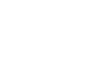How many acres in a mile? - letsdiskussTrishna Dhanda

Self-Starter!!!!! | Posted | Education

# How many acres in a mile?

1
0

Student | Posted

Acre is a term which is used as a measuring tool for measuring the area of the land. An acre is likely to be measured in all shapes like rectangles, circles etc. An anre has a length and width by which the area of the land is measured. If we talk about one square mile, it is 640 acres. There are basically 2 types of acre lands, residential and commercial. On the residential land, the houses, flats and so are built and on the commercial land is owned majorly by the government or the private entities for building malls etc.0
0

Writer | Posted

Do you know what an acre is? Well, an acre is a unit used to measure the area. For example, it is used for measuring the tract of land. Are you aware of how an acre was defined in the Middle ages? The Middle Ages used to define an acre as the amount of land one man and an ox could plow in one day. But what if your ox is feeling lethargic or unwell. But nothing to worry about now as the method has become outdated now.

Acre to mile conversion or mile to acres conversion- Both are required

Suppose you want to purchase a particular property that is situated over certain acres of land. This is the time when you need to know the acres calculation to determine the price of the land.

Are you a student? Then the study of these unit conversations is also very crucial for the ease of cracking related questions.Image Source: Google

Acres to mile conversion

You need to know the value of the area and then divide it by 640. Say, for example, there is one acre of land, then the square mile will be 0.00156.

How many miles in an acre?

Well, 1 acre=0.00156 sq miles.

Mile to acre conversion

The process to convert mile to acre is just the opposite of the process of converting acres to miles. You need to be aware of how many square miles are there. Then multiply the square miles with 640. Suppose there is 1 square mile, then it means there are 640 acres of land.

How many acres in a mile?

It can be concluded that 1 sq mile=640acres.

0
0

translator | Posted

Acre is a unit of area  used for measuring large area of land. An acre can be measured in any shape, from rectangles to circles. It can have any length and width so long as the total area of land is 43,560 square feet because 1 acre is equal to 43,560 sq.ft.

Acre=66 feet × 660 feet = 43,560 square feet

One mile = 5280 feet

one sq. mile = 5280 * 5280 = 27878400 square feet

one sq.mile =27878400 / 43560 = 640 acres0
0